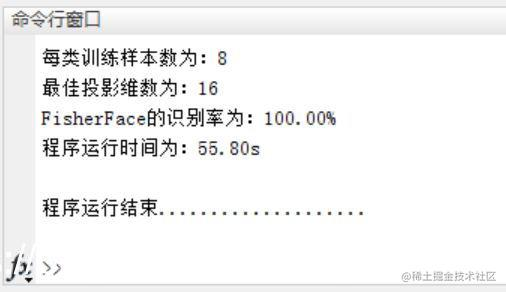# 【人脸识别】基于matlab PCA+LDA人脸识别【含Matlab源码 680期】

## 一、简介

1 PCA
1.1 数据降维

1.2 PCA：目的是降维，降维的实际原理是最大化目标函数（数据投影后的方差最大）

(1)假设有m个n维样本： {Z1,Z2,…,Zm}
(2)样本中心 u 为： 所有样本观测值之和/(mxn)
(3)去中心化后，得到矩阵 {X1,X2,…,Xm}={Z1-U,Z2-U,…,Zm-U}
(4)记含有n个元素的向量W,则样本X1在w方向上的投影为二者内积 X1 . W
(5)PCA的目标函数为最大化投影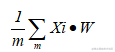（1）构建拉格朗日算子，求导为0，解得投影最大的向量为特征值最大对应的特征向量。

（2）SVD奇异值分解：降维只需求出右奇异矩阵，即AA(T)的特征向量，不需要求A的协方差矩阵。对内存友好。

1.3 基于PCA的人脸识别
（1）基于人脸样本库，例如现实中人脸拍照（银行、车站）等数据采集方法，建立人脸库。
（2）求取训练人脸库的协方差阵的特征值和特征向量。
（3）对于需要判别的人脸，判断其在特征向量上的投影与哪个训练样本的投影最接近。

！！！注：：需要注意，协方差矩阵是维度之间的协方差，故是nxn维，但是在实际应用时，例如图像降维（假设一幅图像有200*10个像素，有100幅图像），一个像素就是一个维度，则原本协方差阵XX’为 （2000x100）x (100x2000)维，计算机存储计算消耗过大，此时可以考虑使用替代矩阵P=X’X(100x2000)x(2000x100)替代：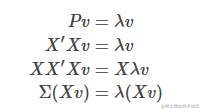P的特征值即原始协方差阵的特征值，P的特征向量左乘数据矩阵即为原始协方差阵的特征向量。

2 LDA
LDA：线性判别分析，也称为Fisher线性判别，是常用的降维技术。

LDA降维后的维度是直接和类别的个数相关的，与数据本身的维度没关系，比如原始数据是n维的，一共有C个类别，那么LDA降维之后，维数取值范围为（1，C-1），举个例子，假设图像分类，两个类别正例反例，每个图像用10000维特征表示，那么LDA之后，就只有1维特征，并且这维特征的分类能力最好。

2.2 LDA数学表示：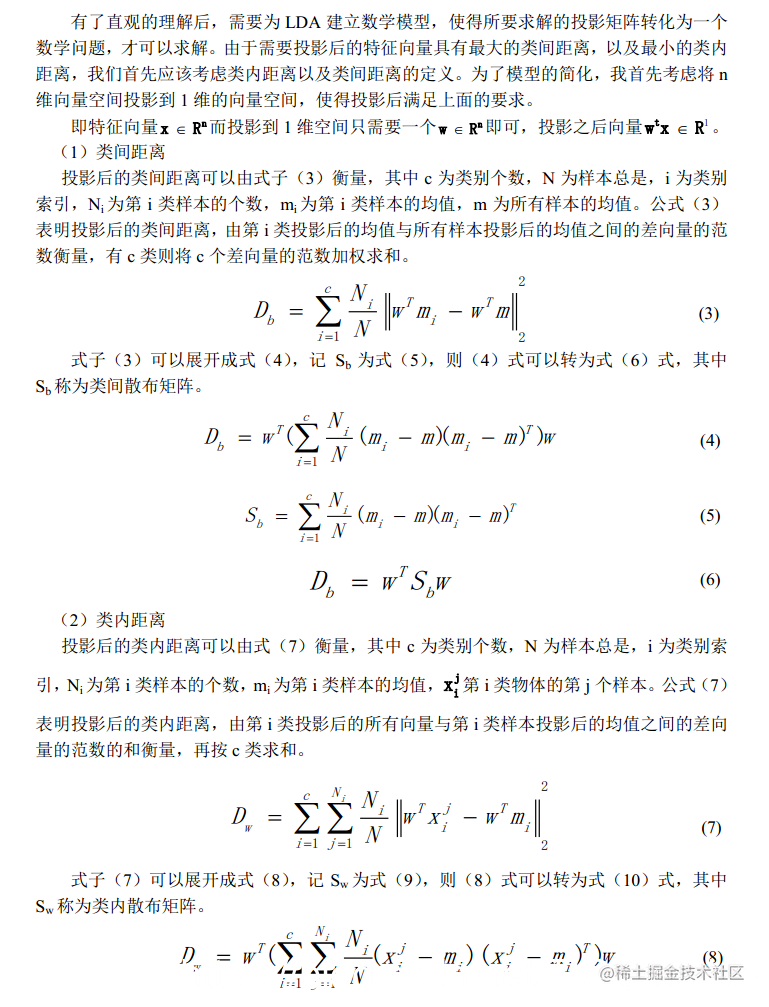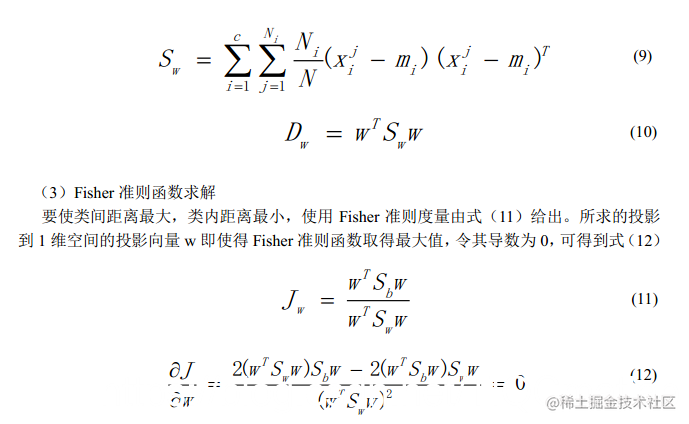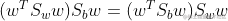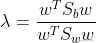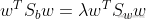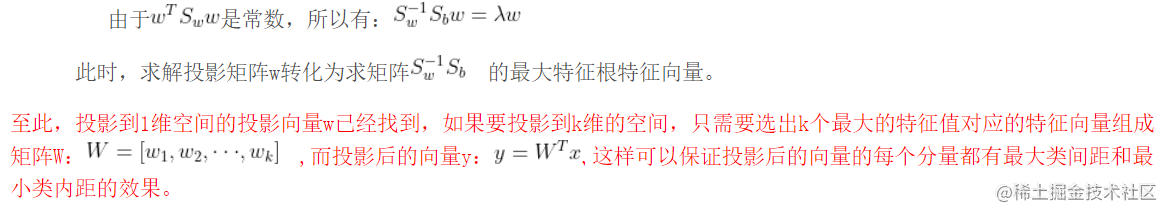## 二、源代码

``````clear all
clc
close all
start=clock;
sample_class=1:40;%样本类别
sample_classnum=size(sample_class,2);%样本类别数
fprintf('程序运行开始....................\n\n');

for train_samplesize=3:8;
train=1:train_samplesize;%每类训练样本
test=train_samplesize+1:10;%每类测试样本

train_num=size(train,2);%每类训练样本数
test_num=size(test,2);%每类测试样本数

%读取训练样本

%先使用PCA进行降维
[newsample base]=pca(allsamples,0.9);
%计算Sw,Sb
[sw sb]=computswb(newsample,sample_classnum,train_num);

%读取测试样本
best_acc=0;%最优识别率
%寻找最佳投影维数
for temp_dimension=1:1:length(sw)
vsort1=projectto(sw,sb,temp_dimension);

%训练样本和测试样本分别投影
tstsample=testsample*base*vsort1;
trainsample=newsample*vsort1;
%计算识别率
accuracy=computaccu(tstsample,test_num,trainsample,train_num);
if accuracy>best_acc
best_dimension=temp_dimension;%保存最佳投影维数
best_acc=accuracy;
end
end
%---------------------------------输出显示----------------------------------
fprintf('每类训练样本数为：%d\n',train_samplesize);
fprintf('最佳投影维数为：%d\n',best_dimension);
fprintf('FisherFace的识别率为：%.2f%%\n',best_acc*100);
fprintf('程序运行时间为：%3.2fs\n\n',etime(clock,start));
end
function [newsample basevector]=pca(patterns,num)
%主分量分析程序，patterns表示输入模式向量，num为控制变量，当num大于1的时候表示
%要求的特征数为num，当num大于0小于等于1的时候表示求取的特征数的能量为num
%输出：basevector表示求取的最大特征值对应的特征向量，newsample表示在basevector
%映射下获得的样本表示。
[u v]=size(patterns);
totalsamplemean=mean(patterns);
for i=1:u
gensample(i,:)=patterns(i,:)-totalsamplemean;
end
sigma=gensample*gensample';
[U V]=eig(sigma);
d=diag(V);
[d1 index]=dsort(d);
if num>1
for i=1:num
vector(:,i)=U(:,index(i));
base(:,i)=d(index(i))^(-1/2)* gensample' * vector(:,i);
end
else
sumv=sum(d1);
for i=1:u
if sum(d1(1:i))/sumv>=num
l=i;
break;
end
end
for i=1:l
vector(:,i)=U(:,index(i));
base(:,i)=d(index(i))^(-1/2)* gensample' * vector(:,i);
end
end
%这个函数用来读取样本。
%输出为样本矩阵
allsamples=[];
[rows cols]=size(image);%获得图像的行数和列数
for i=classnum
for j=num
b=a(1:rows*cols);
b=double(b);
allsamples=[allsamples;b];
end
end

## 三、运行结果